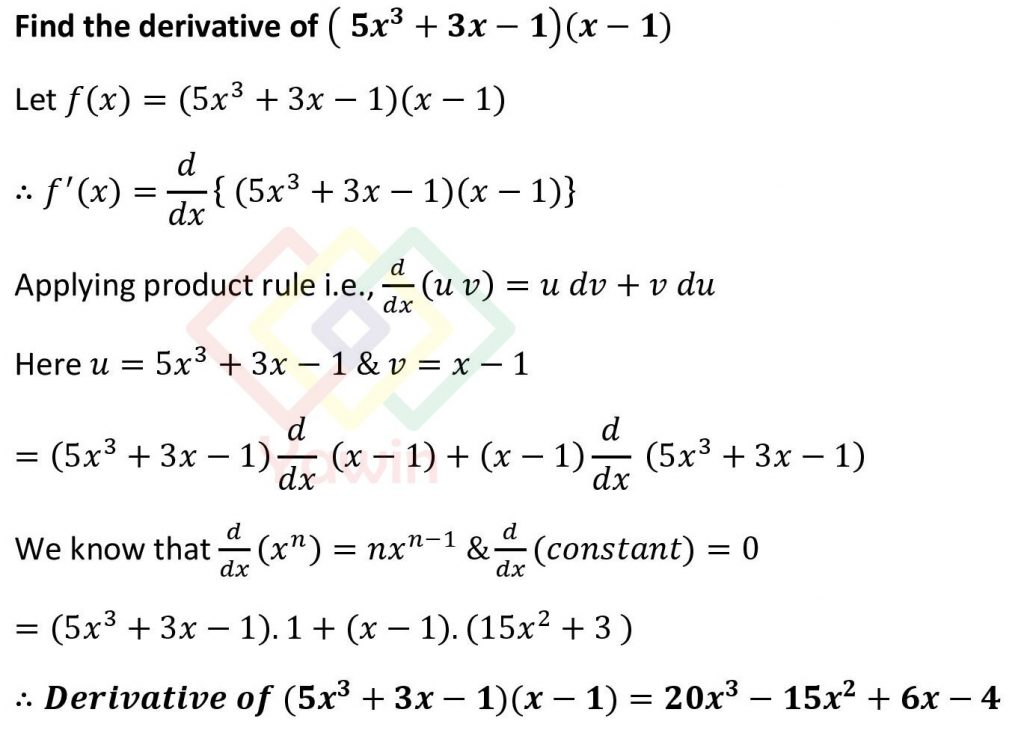Find the derivative of (5x^3+3x-1)(x-1)

## Problem## Question

Find the derivative of (5x^3+3x-1)(x-1)